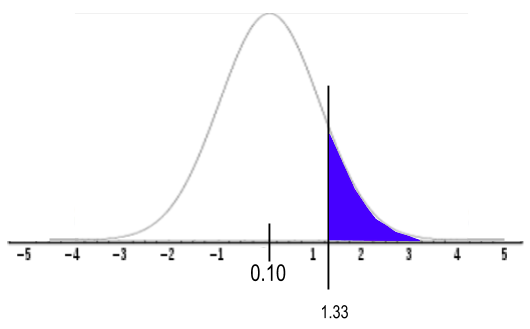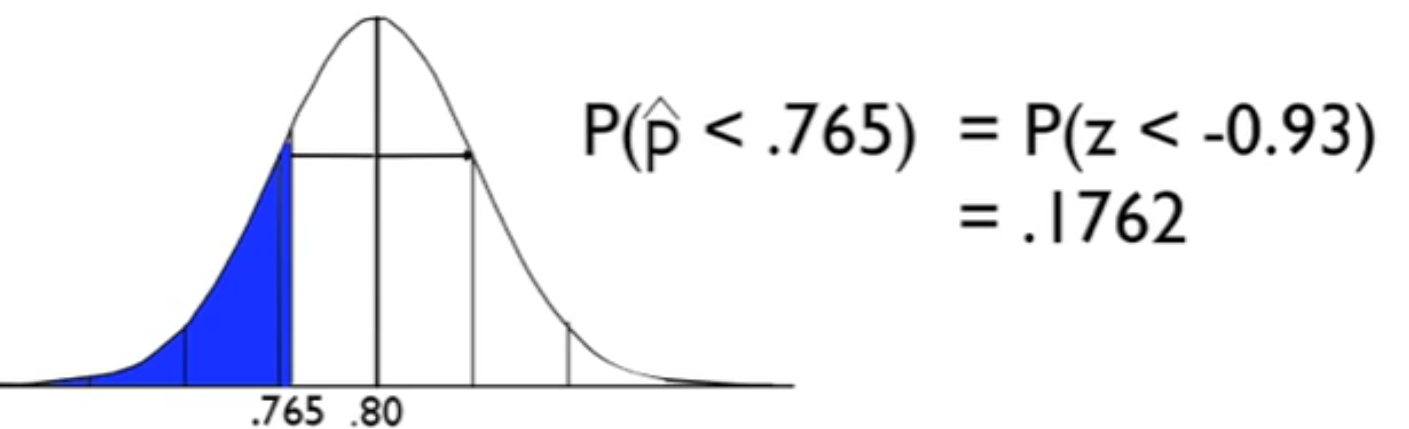+

# Z-Test for Population Proportions

Author: Sophia Tutorial
##### Description:

Calculate z-statistic of a population proportion.

(more)

Sophia’s self-paced online courses are a great way to save time and money as you earn credits eligible for transfer to many different colleges and universities.*

No credit card required

37 Sophia partners guarantee credit transfer.

299 Institutions have accepted or given pre-approval for credit transfer.

* The American Council on Education's College Credit Recommendation Service (ACE Credit®) has evaluated and recommended college credit for 32 of Sophia’s online courses. Many different colleges and universities consider ACE CREDIT recommendations in determining the applicability to their course and degree programs.

Tutorial
what's covered
This tutorial will cover how to calculate a hypothesis test for population proportions. Our discussion breaks down as follows:
1. Calculating a Z-Test for Population Proportions
2. Conducting a Z-Test for Population Proportions

## 1. Calculating a Z-Test for Population Proportions

A hypothesis test for population proportions is a hypothesis test where we compare to see if the sample proportion of "successes" differs significantly from a hypothesized value that we believe is the population proportion of "successes." The type of data that is collected for a population proportion is qualitative data.

big idea
This is also known as a z-test for population proportions

When calculating a z-test for population proportions, you need the following information:

• Population proportion of successes (p)
• Population proportion of failures (1-p = q)
• Sample proportion of successes (p̂)
• Sample size (n)

This information will be plugged into the formula for a z-statistic of population proportions:

formula
Z-Statistic for Population Proportionshint
You can also find this probability using technology.

### IN CONTEXT

Approximately 10% of the population is left-handed, with a standard deviation of 3.13%. Of 100 randomly selected people, 14 claimed to be left-handed.

Find the z-test statistic for this data set.

This type of data is qualitative data; people are answering either yes or no. They're either left-handed or not left-handed. We're placing the answers into categories, which is why it's also called categorical data.

Since we know the population standard deviation, we can use the formula for population proportions to find the z-score. We have p-hat, which is the proportion of successes from our sample. In this case, a success is being left-handed, which is 14 out of 100, or 14%, or 0.14. Now, p is the population number proportion of successes, which is 10%, or 0.10. Then, we have q, which is the complement to p. This tells us that for people who are right-handed, there would be 90%, or 0.90. Our sample size was 100.

Let's go ahead and calculate the z-score.We've got our 14% minus the population proportion of 10%. We're going to calculate divide by the standard error, so the square root of 0.10 times 0.90 all over our sample size of 100. We end up getting a z-test statistic of 1.33.

We can use a normal distribution because we know the population standard deviation. This distribution is centered at 10%. Our sample rendered 14% of people being left-handed, which was 1.33 standard deviations above the mean.term to know

Hypothesis Test for Population Proportions
A hypothesis test where we compare to see if the sample proportion of "successes" differs significantly from a hypothesized value that we believe is the population proportion of "successes."
Z-test for Population Proportions
A type of hypothesis test used to test an assumed population proportion.

## 2. Conducting a Z-Test for Population Proportions

When running a hypothesis test for population proportions, the same four parts apply every time:

Step 1: State the null and alternative hypotheses
Step 2: Check the conditions necessary for inference
Step 3: Calculate the test statistic and the p-value
Step 4: Compare your test statistic to your chosen critical value, or your p-value to your chosen significance level. Based on how they compare, state a decision about the null hypothesis and conclusion in the context of the problem.

EXAMPLE

Let's look at a situation that would require proportions.

A popular consumer report reported that 80% of all supermarket prices end in the digits 9 or 5. Suppose you review a random sample of 115 items to check it against the consumer report, and you find that only 88 items end in 9 or 5. That's less than 80%.

The question that we need to consider is this significantly less than 80%? Is this evidence that, in fact, less than 80% of all items at the supermarket have a price ending in 9 or 5?

step by step
Step 1: State the null and alternative hypothesis.

Your null hypothesis is the "nothing's going on" hypothesis. In this case, it's the "there is no reason to disbelieve the consumer report" hypothesis. Rewrite it as p equals 0.8. The true proportion of all prices ending in 9 or 5 is 80% at the supermarket.

Conversely, the alternative hypothesis suspects that something is amiss, that it is actually less than 80%. We are going to say that p, the true proportion of prices ending in 9 or 5, is below 80%.

In this problem, choose a significance level of 0.10. With the decision rule, if the p-value is less than 0.10, you’ll reject the null hypothesis in favor of the alternative.Step 2: Check the conditions necessary for inference.

You should be familiar with the conditions: randomness, independence, and normality. Look at them one at a time.

Criteria Description
Randomness How were the data collected?

The randomness should be stated somewhere in the problem. Think about the way the data was collected
Independence Population ≥ 10n

Make sure that the population is at least 10 times the size of the sample because you're sampling without replacement.
Normality np ≥ 10 and nq ≥ 10

Because you're using the sampling distribution of p-hat instead of x-bar, there are different conditions for normality. Use the conditions np is at least 10 and nq is at least 10. We can't use the central limit theorem here because this is not the sampling distribution of x-bar. It's the sampling distribution of p-hat, sample proportions.

Going back to the example:

• Randomness: In the problem, it does say that the items were randomly selected, so the simple random sample condition is acceptable.
• Independence: Assume the independence piece--that the population of all items at the grocery store is at least 1,150. That seems reasonable.
• Normality: You know what n is, and you know what p is. So, p is the value from the null hypothesis--it's the 80% that you're believing is the center of the distribution; n was the sample size, 115. Multiply 0.80 times 115 to get 92 for n times p. That's greater than 10. In addition, you get 23 for n times q, which is also greater than 10.The sampling distribution of sample proportions is going to be of an approximately normal sampling distribution.

All three conditions have been checked, and we're good to go.

Step 3: Calculate the test statistic and calculate the p-value based on the normal sampling distribution.

Now you can perform the z-test for population proportions. It's going to be the statistic (88 over 115) minus the hypothesized parameter (0.80) over the standard error. The standard error in this case is the square root of p times q divided by n.When you evaluate the fraction, you get a z-score of negative 0.93. Then, you can find negative 0.93 on the normal distribution that is the sampling distribution for p-hat, and find the tail probability by using a normal z-table.The probability that your sample proportion would be less than the one that you got, the 88 out of 115, is equals to the probability that the z-statistic would be less than negative 0.93.

You can find that area using the normal table, and you get 0.1762, or about 18% of the time. This means that if the null hypothesis was true and this distribution was really centered at 0.8, meaning the true proportion of prices ending in 9 or 5 was 0.8, you would find something at least as low as we got about 18% of the time.

Step 4: Compare your test statistic to your chosen critical value or your p-value to our chosen significance level.

Based on how your p-value compares to your chosen significance level, which you may recall was 0.10, you're going to make a decision about the null hypothesis and state the conclusion. In your case, 0.1762 is greater than 0.10. Your decision, then, is that you fail to reject the null hypothesis. The conclusion is that there's not sufficient evidence to conclude that less than 80% of supermarket prices end in 9 or 5. You don't have strong enough evidence to reject the claim of the consumer report.

• Comparison: 0.1762 > 0.10
• Decision: Fail to reject the null hypothesis
• Conclusion: There is insufficient evidence to conclude that less than 80% of supermarket prices end in 9 or 5.

summary
The steps in any hypothesis test are always the same. You start by stating your null and alternative hypotheses, which is where you would also state your alpha level. Next, state and verify the conditions. Calculate the test statistic and the p-value. Finally, based on your p-value, compare it to your alpha level and make a decision about the null hypothesis and state it in the context of the problem. In this case, we did a z-test for population proportions, and it's analogous to any other hypothesis tests that you do. The only thing that you changed was how you verified the normality condition, because you needed np to be at least 10 and nq to be at least 10.

Good luck!

Source: Adapted from Sophia tutorial by Jonathan Osters.

## Z-Tables

of
Terms to Know
Hypothesis Test for Population Proportions

A hypothesis test where we compare to see if the sample proportion of "successes" differs significantly from a hypothesized value that we believe is the population proportion of "successes."

Z-Test for Population Proportions

A type of hypothesis test used to test an assumed population proportion.

Formulas to Know
z-statistic of ProportionsRating